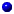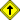Introduction
Overview
History
Books
Tutorials

3D on the Web
Pure Java
Java3D
Flash
VRML
Other

Concepts
The hardest part about 3D graphics knowing where to start. There are so many aspects to it all that beginners are often bewildered. They don't know where to start, what's important, or what can be set aside until later. On this page I try to discuss the pieces of 3D technology in a way that helps map out what the learning process show be. I go into detail on other pages.IntroductionReturn to top of document

### Introduction

3D imaging starts with creating a model (a mathematical description of the objects in a scene) and ends with converting these models to visual images (rendering). A variety of techniques and tools are available for modelling and rendering 3D images. These are discussed in other sections of this site.

But there's an aspect of modelling that often escapes a beginner's attention - one that explains why 3D books and tutorials always seem to start out with discussions of mathematics.

From a viewer's perspective, all 3D images simply consist of surfaces. What goes on beneath the surface doesn't affect the way that the objects appear. Surfaces of real-life objects tend to have lots of curves and irregular shapes - shapes which cannot be easily described by simple equations. This makes it very difficult to model object surfaces mathematically by simply using equations. Instead, 3D graphics designers have found that breaking the surfaces down into smaller areas can enable the designers to work with objects of almost any complexity.

In particular, object surfaces are represented in models by an interconnecting mesh of polygons. More specifically, the polygons most frequently used used to represent surfaces are triangles. Again, the approach of using polygons (triangles) is utilized because mathematical techniques and software algorithms exist which can efficiently manipulate polygon (triangle) meshs (surfaces). Such techniques do not generally exist for working with complex mathematical surface equations.

There are some specific exceptions to the use of triangles as the polygon of choice, but virtually the entire 3D industry revolves around modelling of surfaces using triangles. This adoption of triangles as the polygon of choice is also used by the PC graphics accelerator cards. Graphics accelerator cards are designed to perform operations on triangles at blazing speeds.

The concept of representing 3D surfaces with polygons (triangles) for ease of computation is very powerful and forms the basis for most of the 3D acceleration hardware cards that you can buy for your PC display system. We will be discussing the various techniques for handling polygons, including manipulations such as translation, rotation, and scaling. We'll also discuss shading polygons - giving them visual properties such as colors, textures, and lighting (reflections and refaction). Shading of all polygons in a 3D scene is typically called rendering.

It may not be obvious but a key constraint placed on polygons used in 3D models is co-planarity, that is, all points on the polygon are co-planar. This greatly simplifies the code needed for manipulating and shading polygons. If the polyons used to create a surface were not co-planar, the mathematics needed to work with them would be considerable more difficult because the equations describing the points on the plane would be more complex non-linear equations rather than simpler linear equations. Linear equations are much simpler to program with software than non-linear equations and also compute faster.

Triangle polygons, in addition to providing the co-planar surface, have the added benefit of being the simplest polygon of all - needng only 3 points (each point is called a vertex) to define them, The simplicity of working with triangles will become more even more apparent are we proceed through these tutorials.

Now that the triangle is established as the polygon (surface) of choice, we can begin to focus on the mathematics needed to manipulate and shade them. First, let's make this very important distinction about the mathematics needed to work with triangles:

• The math used to work with triangles is high school math - trigonometry, geometry and algebra. You won't need your college calculus or other high-level math classes to become a 3D practioner.

I'm not saying that 3D math is trivial, but I want to make sure that budding 3D graphics designers are not put off by concerns that the math will be too complicated. The mathematics of 3D design are well within the ability of most programmers to learn.

You will need to become familiar with one math concept which you may or may not have been exposed to in high school - vectors. If you've watched the TV weatherman, then you've probably seen vectors and didn't know it. When the weatherman shows a map of wind speed/direction, it's often a geography map covered with many small arrows pointing in the direction the wind is blowing. The length (or sometimes thickness) of the arrows indicates how fast the wind is blowing.

These arrows are vectors - information about direction and magnitude of a parameter (wind, in this case). We'll talk much more about this in the math section.

But, to show why math (and vectors) are important consider this simple, but powerful example. If you wanted to display 10 walls on a PC, lined up one behind the other, you have two choices.

You can tell the computer to draw each of the walls one at a time, writing each wall in front of the other - starting at the farthest wall and continuing to the closest wall. This works just fine but when you're done all you see on the screen is the image of the nearest wall. The walls 'behind' the closest wall cannot be seen. (I'm assuming that the viewpoint of the observer is in front of the first wall).

If you had a thousand walls one behind the other, you can appreciate that it would take a lot of computer time to draw all thousand walls - only to end up with a single wall that you can see.

Despite the speed and power of today's computers, it is easy for a 3D graphics designer to creates scenes more complex than a computer can create in real time. This is the major reason why all of the various software techniques and algorithms exist - to get around the hardware limitations that face 3D graphics designers. Future hardware and software may lighten this problem, but solutions are not expected in the near future. Today, a great deal of effort goes into developing techniques which work around the limitations in hardware speeds - of providing real-time images with the fewest possible trade-offs in image photo-realism. Throughout these tutorial pages you'll see comments referring to the speed of rendering as a major factor in choosing one technique over another.

The second option you have in speeding up the displaying of the scene of a thousand walls is to determine which wall can be seen and only draw (display) that wall - cutting your drawing time down by orders of magnitude. Computers are much faster at performing the calculations than they are at writing information to a display, so the more you can "crunch" the data first, the more you will improve display time.

The particular vector math operation that can help us out in this expample is vector multiplication. Remember that a vector has only two properties - magnitude and direction. It has no position in space.

However, by treating the sides of a triangle (polygon) as though they were vectors we can use vector multiplcation to determine whether one polygon (wall) is in front of another. Polygons (walls) behind another polygon (wall) do not need to be drawn because they cannot be seen. In this example we would have to draw only one of the thousand walls, a huge saving in rendering time. As we will see, it only takes a few lines of code to perform the vector multiplication - not very complicated at all.

We'll go into much more detail on vectors and applying vector math to triangles (polygons) in the math section of this site. For now, in an attempt to emphasize the importance of learning and understanding vector math, I simply wanted to show that 3D graphics rendering time can be greatly reduced by the application of some very straight-forward vector math.

All of this - with just the use of high school mathematics!